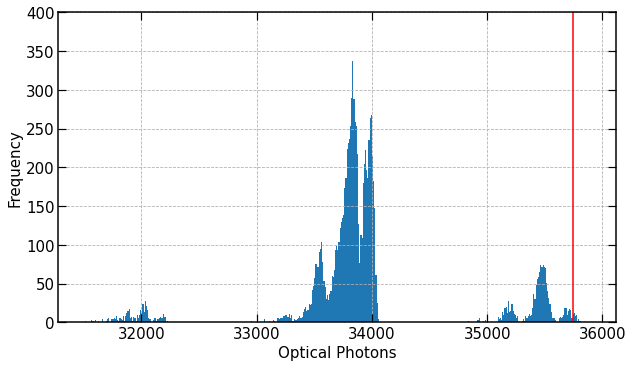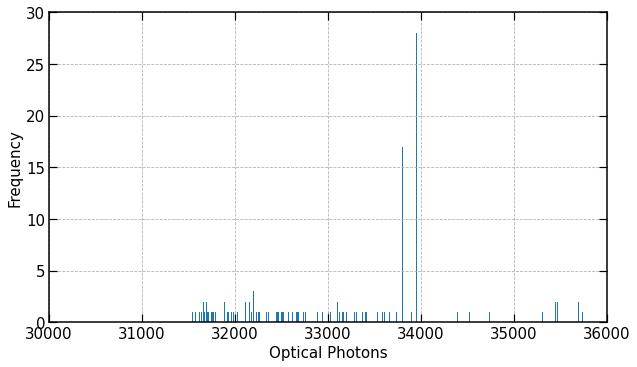# Counting optical photons from scintillation events

To convince myself that I am implementing non-proportional scintillation yield correctly I plan on replicating figure 2 in The light yield nonproportionality component of scintillator energy resolution | IEEE Journals & Magazine | IEEE Xplore by counting optical photons produced by electrons which are part of a chain where the primary at some point underwent photoelectric absorption. Results are separated based on final interaction type after the simulation is complete. For this I want to make sure that I am even counting optical photons correctly.

To build up to this I setup a linear yield case and defined the resolution scale to be zero so that I could observe the total yield lines corresponding to which shells that the primary gamma interacted when scattering or being absorbed.

However, I recorded more photons in some cases than should have been possible for my setup (yield=54 photon/keV, resolutionscale=0) as well as not seeing discrete linesRedline show the maximum number of optical photons I think should ever be produced, 54*(primary energy=662keV), my code used to snag the output was

``````G4bool ScintillatorSD::ProcessHits(G4Step *aStep, G4TouchableHistory *ROhist)
{
const G4String& PostProcessName =
aStep->GetPostStepPoint()->GetProcessDefinedStep()->GetProcessName();
const G4double& DeltaEnergy =
aStep->GetDeltaEnergy();

G4Track *track = aStep->GetTrack();

auto secTrack = aStep->GetSecondaryInCurrentStep();
G4double secSize = (*secTrack).size();

G4ParticleDefinition* particle = track->GetDefinition();
const G4String& particleName = particle->GetParticleName();

// Call analysis manager
G4CsvAnalysisManager *man = G4CsvAnalysisManager::Instance();

// If the particle is a primary, save interaction type, this is how I sort
// my data later.
if (track->GetTrackID() == 1) {
if(DeltaEnergy < 0) {
// Save event ID
man->FillNtupleIColumn(0, 0, G4RunManager::GetRunManager()->GetCurrentEvent()->GetEventID());
// If Photoelectric save 1
if (PostProcessName == "phot") {
man->FillNtupleIColumn(0, 1, 1);
}
// If Not photoelectric save 0
else{
man->FillNtupleIColumn(0, 1, 0);
}
}

// Not a primary, check if it is an electron
} else if (particleName == "e-") {
// check if there was any energy loss
if(DeltaEnergy < 0) {
// Now start counting the number of secondary optical photons
G4int numoptical = 0;
// Loop through all secondaries
for (int i=0; i<secSize; i++){
G4String secondaryName = (*secTrack)[i]->GetDefinition()->GetParticleName();
// Increment counter if secondary is an optical photon
if (secondaryName=="opticalphoton"){
numoptical = numoptical + 1;
}
}
// save event ID and number of optical photons
man->FillNtupleIColumn(1, 0, G4RunManager::GetRunManager()->GetCurrentEvent()->GetEventID());
man->FillNtupleDColumn(1, 1, numoptical);
// Kill secondary electron
//track->SetTrackStatus(fStopAndKill);
}
}
return true;
}
``````

Now if I kill my electrons after their first step, I do see discrete lines as I expect, maybe because what I see are the results of photons produced by electrons which gave up energy in one stepSo my question is this, am I double counting optical photons causing the yield to not be located at discrete locations, or is this a product of how the optical photons are produced in Geant4?

1 Like

This may be because, when the mean number of optical photons in a step is <= 10, the actual number is drawn from a Poission distribution.

https://geant4.kek.jp/lxr/source/processes/electromagnetic/xrays/src/G4Scintillation.cc#L422

``````  if(MeanNumberOfPhotons > 10.)
{
G4double sigma = ResolutionScale * std::sqrt(MeanNumberOfPhotons);
fNumPhotons = G4int(G4RandGauss::shoot(MeanNumberOfPhotons, sigma) + 0.5);
}
else
{
fNumPhotons = G4int(G4Poisson(MeanNumberOfPhotons));
}
``````

RESOLUTIONSCALE doesn’t affect this.

2 Likes

Thanks for the reply! I do observe that the steps a electron takes where the released number of optical photons were less than 10 occurs on the order of 1e1, so this seems the likely answer for why I observe the deviations. And now I can have a bit more confidence that I am counting my optical photons correctly!

Hey! I was also simulating a scintillator but my physics processes are only printing Transportation and OpAbsorption. Can tell where it is wrong ?

This topic was automatically closed 7 days after the last reply. New replies are no longer allowed.